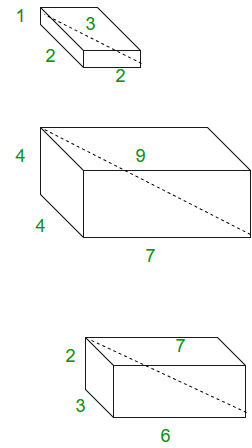Given four points, check whether they form Pythagorean Quadruple.
It is defined as a tuple of integers a, b, c, d such that. They are basically the solutions of Diophantine Equations. In the geometric interpretation it represents a cuboid with integer side lengths |a|, |b|, |c| and whose space diagonal is |d| .The cuboids sides shown here are examples of pythagorean quadruples.
It is primitive when their greatest common divisor is 1. Every Pythagorean quadruple is an integer multiple of a primitive quadruple. We can generate the set of primitive pythagorean quadruples for which a is odd can be generated by formula :

a = m2 + n2 – p2 – q2,
b = 2(mq + np),
c = 2(nq – mp),
d = m2 + n2 + p2 + q2

where m, n, p, q are non-negative integers with greatest common divisor 1 such that m + n + p + q are odd. Thus, all primitive Pythagorean quadruples are characterized by Lebesgue’s identity.

(m2 + n2 + p2 + q2)2 = (2mq + 2nq)2 + 2(nq – mp)2 + (m2 + n2 – p2 – q2)m2 + n2 – p2 – q2

## C++

 `// C++ code to detect Pythagorean Quadruples. ` `#include ` `using` `namespace` `std; ` ` `  `// function for checking ` `bool` `pythagorean_quadruple(``int` `a, ``int` `b, ``int` `c,  ` `                                        ``int` `d) ` `{ ` `    ``int` `sum = a * a + b * b + c * c; ` `    ``if` `(d * d == sum) ` `        ``return` `true``; ` `    ``else` `        ``return` `false``; ` `} ` ` `  `// Driver Code ` `int` `main() ` `{ ` `    ``int` `a = 1, b = 2, c = 2, d = 3; ` `    ``if` `(pythagorean_quadruple(a, b, c, d)) ` `        ``cout << ``"Yes"` `<< endl; ` `    ``else` `        ``cout << ``"No"` `<< endl; ` `} `

## Java

 `// Java code to detect Pythagorean Quadruples. ` `import` `java.io.*; ` `import` `java.util.*; ` ` `  `class` `GFG { ` ` `  `// function for checking ` `static` `Boolean pythagorean_quadruple(``int` `a, ``int` `b, ` `                                   ``int` `c, ``int` `d) ` `{ ` `    ``int` `sum = a * a + b * b + c * c; ` `    ``if` `(d * d == sum) ` `        ``return` `true``; ` `    ``else` `        ``return` `false``; ` `} ` ` `  `// Driver function ` `    ``public` `static` `void` `main (String[] args) { ` `    ``int` `a = ``1``, b = ``2``, c = ``2``, d = ``3``; ` `    ``if` `(pythagorean_quadruple(a, b, c, d)) ` `        ``System.out.println(``"Yes"``); ` `    ``else` `        ``System.out.println(``"No"` `); ` `         `  `    ``} ` `} ` `// This code is contributed by Gitanjali. `

## Python3

 `# Python  code to detect ` `# Pythagorean Quadruples. ` `import` `math ` ` `  `# function for checking ` `def` `pythagorean_quadruple(a,b, c, d): ` ` `  `    ``sum` `=` `a ``*` `a ``+` `b ``*` `b ``+` `c ``*` `c; ` `    ``if` `(d ``*` `d ``=``=` `sum``): ` `        ``return` `True` `    ``else``: ` `        ``return` `False` ` `  `#driver code ` `a ``=` `1` `b ``=` `2` `c ``=` `2` `d ``=` `3` `if` `(pythagorean_quadruple(a, b, c, d)): ` `    ``print``(``"Yes"``) ` `else``: ` `     ``print``(``"No"` `) ` ` `  `# This code is contributed ` `# by Gitanjali. `

## C#

 `// C# code to detect  ` `// Pythagorean Quadruples. ` `using` `System; ` ` `  `class` `GFG { ` ` `  `    ``// function for checking ` `    ``static` `Boolean pythagorean_quadruple(``int` `a, ` `                            ``int` `b, ``int` `c, ``int` `d) ` `    ``{ ` `        ``int` `sum = a * a + b * b + c * c; ` `        ``if` `(d * d == sum) ` `            ``return` `true``; ` `        ``else` `            ``return` `false``; ` `    ``} ` `     `  `    ``// Driver function ` `        ``public` `static` `void` `Main () { ` `             `  `        ``int` `a = 1, b = 2, c = 2, d = 3; ` `         `  `        ``if` `(pythagorean_quadruple(a, b, c, d)) ` `            ``Console.WriteLine(``"Yes"``); ` `        ``else` `            ``Console.WriteLine(``"No"` `); ` `             `  `    ``} ` `} ` ` `  `// This code is contributed by vt_M. `

## PHP

 ` `

Output:

```Yes
```

References
Wiki
mathworld

Attention reader! Don’t stop learning now. Get hold of all the important DSA concepts with the DSA Self Paced Course at a student-friendly price and become industry ready.

My Personal Notes arrow_drop_upCheck out this Author's contributed articles.

If you like GeeksforGeeks and would like to contribute, you can also write an article using contribute.geeksforgeeks.org or mail your article to contribute@geeksforgeeks.org. See your article appearing on the GeeksforGeeks main page and help other Geeks.

Please Improve this article if you find anything incorrect by clicking on the "Improve Article" button below.

Improved By : vt_m

Article Tags :
Practice Tags :

Be the First to upvote.

Please write to us at contribute@geeksforgeeks.org to report any issue with the above content.